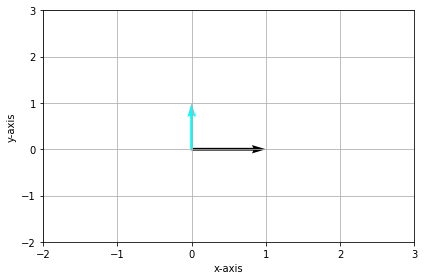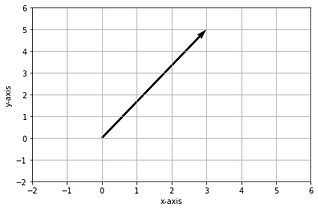By Taaniya Arora, Data Scientist

Photo by Michael Dziedzic on Unsplash

The field of Natural Language Processing involves building techniques to process text in natural language by people like you and me, and extract insights from it for performing a variety of tasks from interpreting user queries on search engines and returning web pages, to solving customer queries as chatbot assistant. The importance of representing every word into a form that captures the meaning of the word and the overall context becomes crucial especially when major decisions are based upon the insights extracted from text on a large scale — like forecasting stock price change with social media.

In this article, we’ll begin with the basics of linear algebra to get an intuition of sof vectors and their significance for representing specific types of information, the different ways of representing text in vector space, and how the concept has evolved to the state of the art models we have now.

We’ll step through the following areas –

• Unit vectors in our coordinate system
• Linear combination of vectors
• Span in vector coordinate system
• Collinearity & multicollinearity
• Linear dependence and independence of vectors
• Basis vectors
• Vector Space Model for NLP
• Dense Vectors

## Unit vectors in our coordinate system

i-> Denotes a unit vector (vector of length 1 unit) pointing in the x-direction

j -> Denotes a unit vector in the y-direction

Together, they are called the basis of our coordinate vector space.

We’ll come to the term basis more in the subsequent parts below.Standard Unit vectors — Image by Author

• Suppose we have a vector 3i+ 5j
• This vector has x,y coordinates : 3 & 5 respectively
• These coordinates are the scalars that flip and scale the unit vectors by 3 & 5 units in the x & y directions respectivelyA vector in 2D X-Y space — Image by Author

## Linear Combination of 2 vectors

If u & v are two vectors in a 2 dimensional space,then their linear combination resulting into a vector l is represented by –

l = x1. u + x2. v

• The numbers x1x2 are the components of a vector x
• This is essentially a scaling and addition operation by x on the given vectors.

The above expression of linear combination is equivalent to the following linear system –

Bx = l

Where B denotes a matrix whose columns are u and v.

Let’s understand this by an example below with vectors u & v in a 2 dimensional space –

``````# Vectors u & v
# The...``````